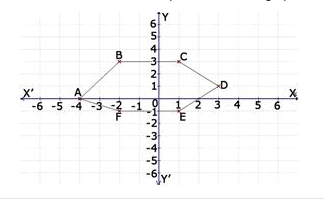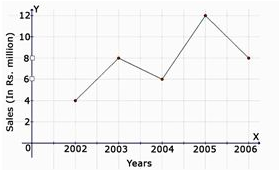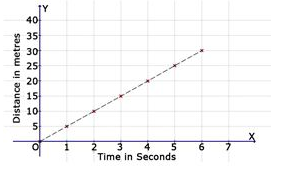Courses

# Test: Introduction To Graphs- 2

## 20 Questions MCQ Test Mathematics (Maths) Class 8 | Test: Introduction To Graphs- 2

Description
This mock test of Test: Introduction To Graphs- 2 for Class 8 helps you for every Class 8 entrance exam. This contains 20 Multiple Choice Questions for Class 8 Test: Introduction To Graphs- 2 (mcq) to study with solutions a complete question bank. The solved questions answers in this Test: Introduction To Graphs- 2 quiz give you a good mix of easy questions and tough questions. Class 8 students definitely take this Test: Introduction To Graphs- 2 exercise for a better result in the exam. You can find other Test: Introduction To Graphs- 2 extra questions, long questions & short questions for Class 8 on EduRev as well by searching above.
QUESTION: 1

### Find the coordinates of the point C from the graph.Solution:

As x-coordinate is 1 unit and y-coordinate is 3 units.

QUESTION: 2

Solution:
QUESTION: 3

### Find the coordinates of the point E from the graph.Solution:
QUESTION: 4

Find the coordinates of the point F from the graph.Solution:
QUESTION: 5

In which quadrant does the point Q (-2, -6) lie?

Solution:

The third quadrant is the quadrant where x and y are negative. Hence (-2,-6) lie in 3rd quadrant

QUESTION: 6

(o, y) are the co-ordinates of a point lying on which of the following?

Solution:

As we don’t have any units for x-coordinate the ,so the point lies on the y-axis.

QUESTION: 7

The line graph shows the yearly sales figure for a manufacturing company. From the graph, what were the sales in 2004?Solution:
QUESTION: 8

The line graph shows the yearly sales figure for a manufacturing company. From the graph, what were the sales in 2002?Solution:
QUESTION: 9

The line graph shows the yearly sales figure for a manufacturing company. From the graph, what were the sales in 2003?Solution:
QUESTION: 10

The line graph shows the yearly sales figure for a manufacturing company. From the graph, what were the sales in 2005?Solution:
QUESTION: 11

The line graph shows the yearly sales figure for a manufacturing company. From the graph, what were the sales in 2006?Solution:
QUESTION: 12

On which axis does the point (0, 5) lie?

Solution:
QUESTION: 13

In which quadrant does the point P (-5, -1) lie?

Solution:

In first quadrant we have (+,+) in second quadrant we have (-,+) in third quadrant we have (-,-) and in 4quadrant we have (+,-)so (-5,-1)lies in 3rd quadrant.

QUESTION: 14

Find the time taken by a body to cover 30 metres.Solution:
QUESTION: 15

Find the time taken by a body to cover 25 metres.Solution:
QUESTION: 16

Find the time taken by a body to cover 20 metres.Solution:
QUESTION: 17

Find the time taken by a body to cover 10 metres.Solution:
QUESTION: 18

Find the time taken by a body to cover 5 metres.Solution:
QUESTION: 19

On which axis does the point (5, 0) lie?

Solution:
QUESTION: 20

The point (0, 4) lies on which of the following:

Solution:

There are no units for x-coordinate and 5 units of y- coordinate . So the point lies on the y-axis.